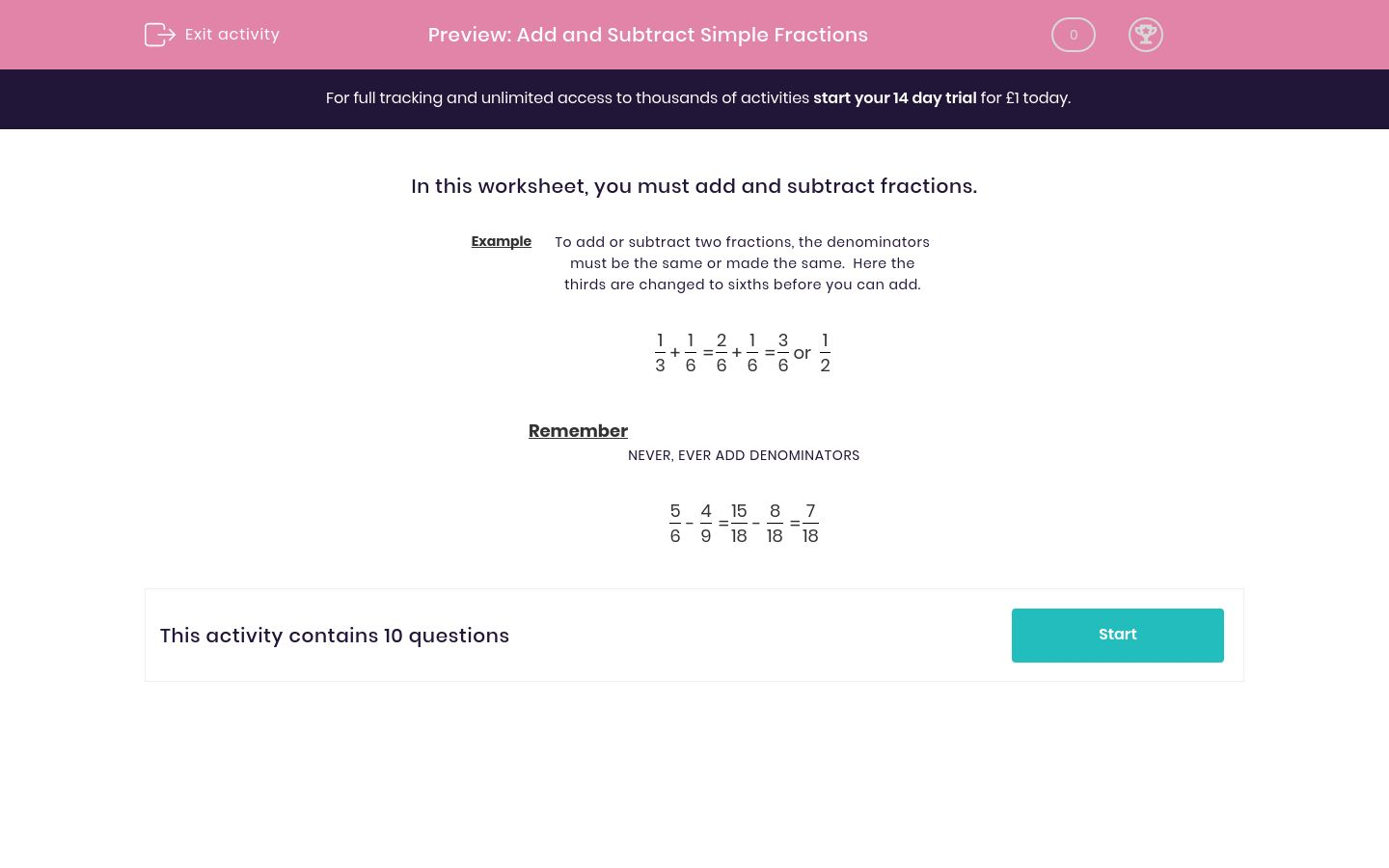# Add and Subtract Simple Fractions

In this worksheet, students improve their skills at adding and subtracting simple fractions.Key stage:  KS 2

Curriculum topic:   Maths and Numerical Reasoning

Curriculum subtopic:   Mixed Problems

Difficulty level:### QUESTION 1 of 10

In this worksheet, you must add and subtract fractions.

Example

To add or subtract two fractions, the denominators must be the same or made the same.  Here the thirds are changed to sixths before you can add.

 1 3
+
 1 6
=
 2 6
+
 1 6
=
 3 6
or
 1 2

Remember

 5 6
-
 4 9
=
 15 18
-
 8 18
=
 7 18
---- OR ----